# Create scatter plots using Python (matplotlib `pyplot.scatter`)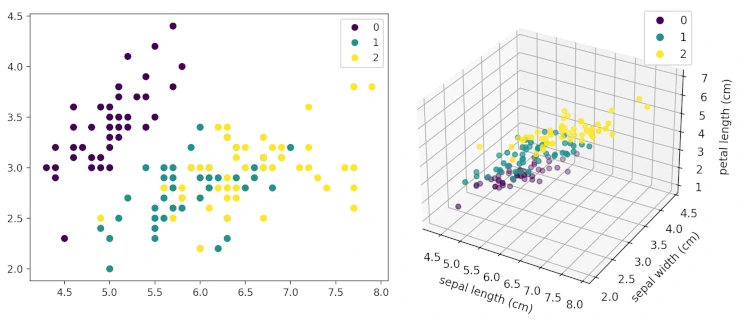## What is scatter plot?

Scatter plot (Scatter graph) represents the plot of individual data points to visualize the relationship between two (2D) or three (3D) numerical variables.

Scatter plots are used in numerous applications such as correlation and clustering analysis for exploring the relationship among the variables. For example, in correlation analysis, scatter plots are used to check if there is a positive or negative correlation between the two variables.

## How to draw a scatter plot in Python (matplotlib)?

In this article, scatter plots will be created from numerical arrays and pandas DataFrame using the `pyplot.scatter()` function available in matplotlib package. In addition, you can also use pandas `plot.scatter()` function to create scatter plots on pandas DataFrame.

### Create basic scatter plot (2D)

For this tutorial, you need to install `NumPy`, `matplotlib`, `pandas`, and `sklearn` Python packages. Learn how to install python packages

#### Get dataset

First, create a random dataset,

``````import numpy as np

x = np.random.normal(size=20, loc=2)
y = np.random.normal(size=20, loc=6)
``````

#### Draw scatter plot

``````import matplotlib.pyplot as plt

plt.scatter(x, y)
plt.title('Basic Scatter plot')
plt.xlabel('x')
plt.ylabel('y')
plt.show()
``````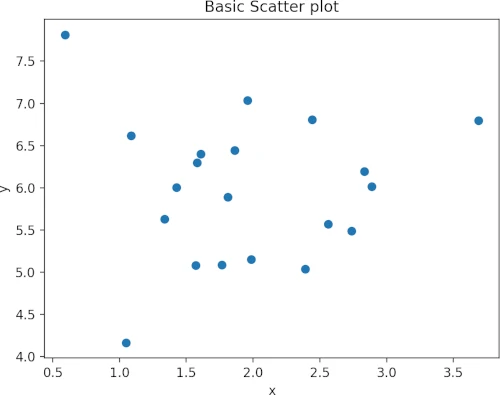The `plt.show()` is necessary to visualize the plot. If you like to save the plot to a file, you need to call `pyplot.savefig()` function. For example to save plot, use the below command,

``````plt.savefig("scatterplot.png", dpi=300, format="png")
``````

Check other parameters for `pyplot.savefig()` here

#### Marker and color

`marker` and `c` parameters are used for changing the marker style and colors of the data points. The default marker style is a circle (defined as `o`)

Change marker and color of the data point,

``````plt.scatter(x, y, marker="s", c="r")
plt.title('Scatter plot with marker and color change')
``````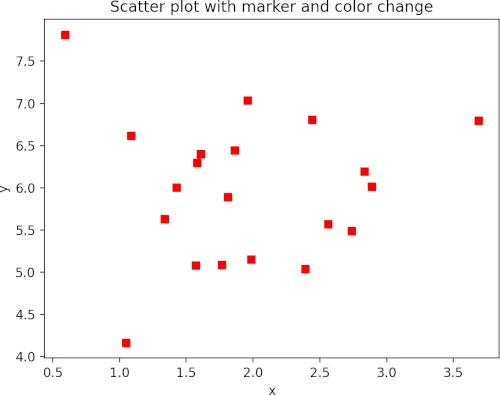#### Markersize and transparency

Change the markersize and transparency of data points using `s` and `alpha` parameters. The `alpha` takes a value between 0 (transparent) and 1 (opaque).

``````plt.scatter(x, y, marker="s", c="r", s=60, alpha=0.5)
plt.title('Scatter plot with markersize and transparency change')
``````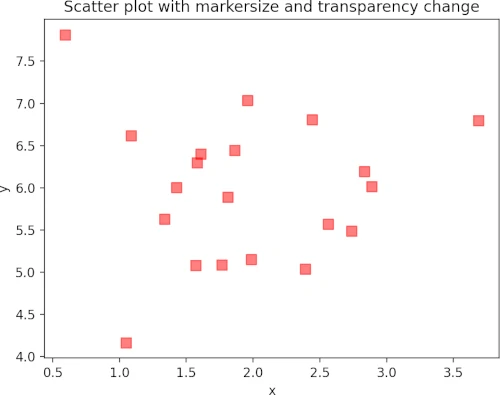#### Colormap

The colormap instance can be used to map data values to RGBA color for a given colormap. The colormap option is provided using the `cmap` parameter. You also need to pass the `c` parameter as an array of floats to draw the colormap.

The default colormap is `viridis`. Get more in-built colormaps here

``````colors = [*range(0, 100, 5)]
plt.scatter(x, y, c=colors, cmap="viridis")
plt.title('Scatter plot with colormap')
plt.colorbar()
``````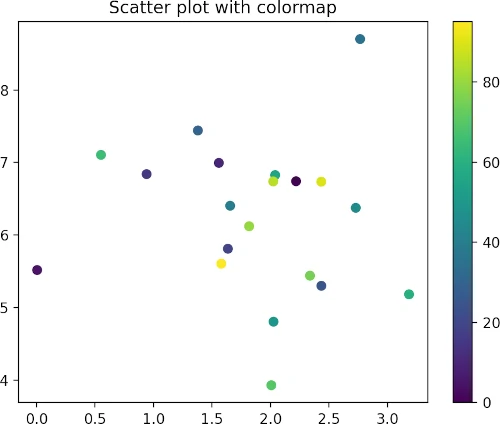To control the starting and end limits of the colorbar, you can pass `vmin` and `vmax` parameters,

``````colors = [*range(0, 100, 5)]
plt.scatter(x, y, c=colors, vmin=10, vmax=90, cmap="viridis")
plt.title('Scatter plot with colormap and limit')
plt.colorbar()
``````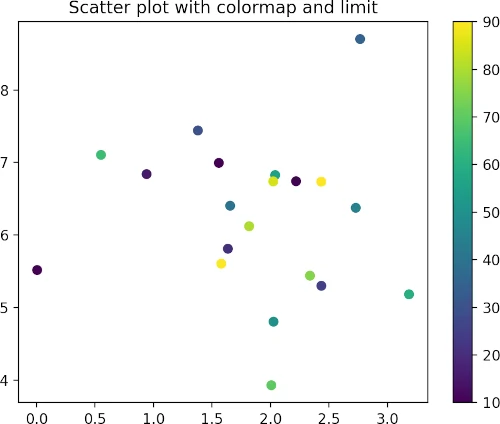#### Add horizontal and vertical lines on the scatterplot

The `pyplot.axhline()` and `pyplot.axvline()` functions can be used to add horizontal and vertical lines along the figure axes, respectively.

For horizontal lines, the position on the y-axis should be provided. Additionally, `xmin` and `xmax` parameters can also be used for covering the portion of the figure.

``````plt.scatter(x, y)
plt.axhline(y=6, color='k', linestyle='dashed')
plt.title('Basic Scatter plot with horizontal line')
``````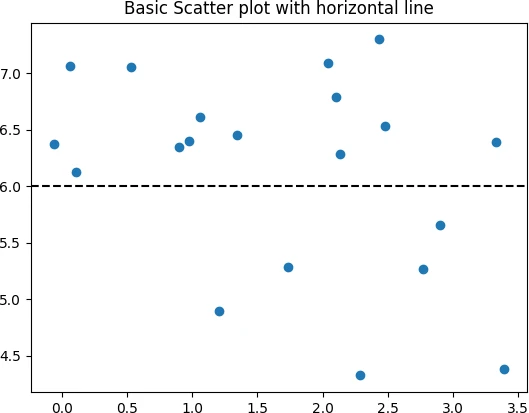For the vertical line, the position on the x-axis should be provided. Additionally, `ymin` and `ymax` parameters can also be used for covering the portion of the figure.

``````plt.scatter(x, y)
plt.axvline(x=2, color='k', linestyle='dashed')
plt.title('Basic Scatter plot with vertical line')
``````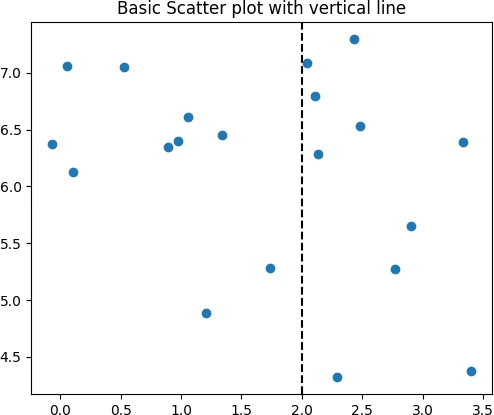#### Markersize based on the size of each data point

Change the sizes of the data points using `s` parameter based on the additional variable of the same length as `x` and `y`,

``````import random

sizes = random.sample(range(1, 100), 20)
plt.scatter(x, y, s=sizes)
``````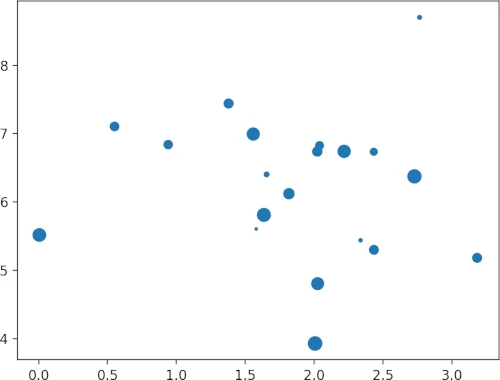#### Compare different scatter plots

You can overlay multiple scatterplots in the same plot for visualizing the different datasets

``````x2 = np.random.normal(size=20, loc=5)
y2 = np.random.normal(size=20, loc=15)

plt.scatter(x, y, label="x-y")
plt.scatter(x2, y2, label="x2-y2")
plt.legend()
``````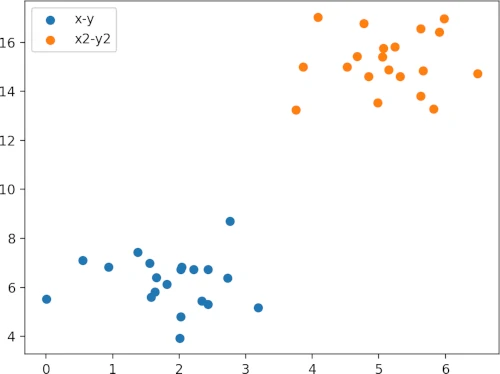#### Side by side subplots

You can create two scatter plots (grid of subplots) within a same figure,

``````fig, (ax1, ax2) = plt.subplots(1, 2)  # 1 row, 2 columns
ax1.scatter(x, y, c='blue')
ax2.scatter(x2, y2, c='red')
ax1.set_xlabel('x')
ax2.set_xlabel('x2')
ax1.set_ylabel('y')
ax2.set_ylabel('y2')
plt.show()
``````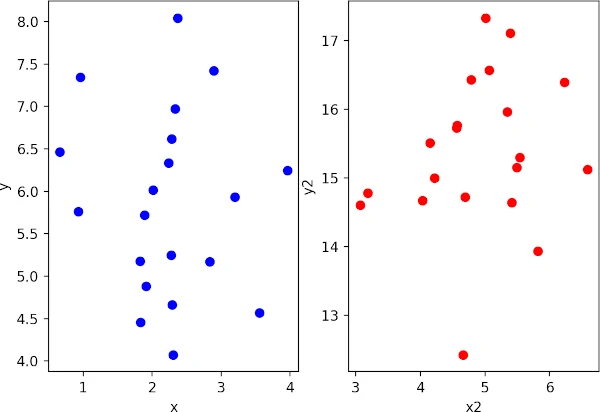Create two scatter plots (grid of subplots) within a same figure with shared axis,

``````fig, (ax1, ax2) = plt.subplots(1, 2, sharey=True)  # 1 row, 2 columns
ax1.scatter(x, y, c='blue')
ax2.scatter(x2, y2, c='red')
ax1.set_xlabel('x')
ax2.set_xlabel('x2')
plt.show()
``````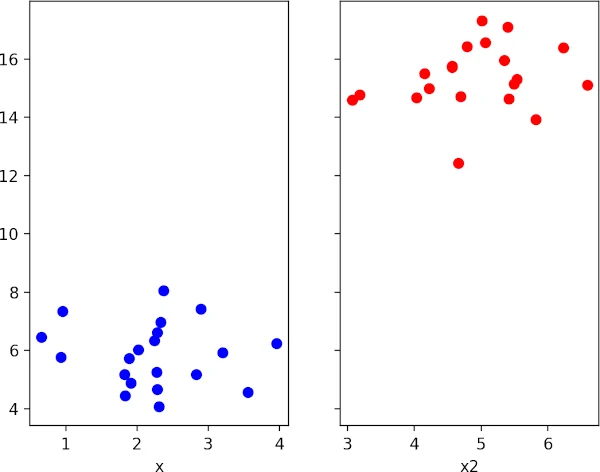### Create scatter plot for multivariate data

The scatter plot can be used for visualizing the multivariate data. I will use the example of the iris dataset which contains the four features, three classes/target (type of iris plant), and 150 observations.

In this example, you will also learn how to create a scatterplot from pandas DataFrame

``````from sklearn.datasets import load_iris
import pandas as pd

# make it as pandas dataframe
df = pd.DataFrame(data=data.data, columns=data.feature_names)
df['target'] = data['target']
# output
sepal length (cm)  sepal width (cm)  petal length (cm)  petal width (cm)  target
0                5.1               3.5                1.4               0.2        0
1                4.9               3.0                1.4               0.2        0

# scatter plot with two features
plt.scatter(df["sepal length (cm)"], df["sepal width (cm)"], c=df["target"])
plt.xlabel('sepal length (cm)')
plt.ylabel('sepal width (cm)')
plt.show()
``````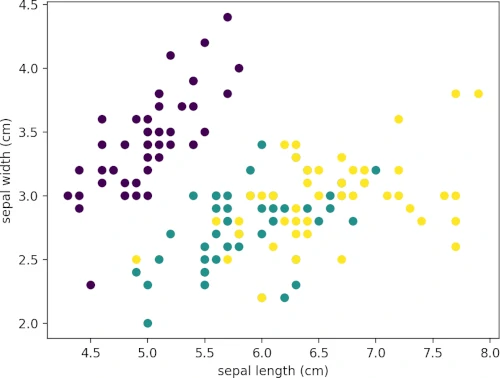``````s = plt.scatter(df["sepal length (cm)"], df["sepal width (cm)"], c=df["target"])
plt.legend(s.legend_elements(), list(set(df["target"])))
plt.show()
``````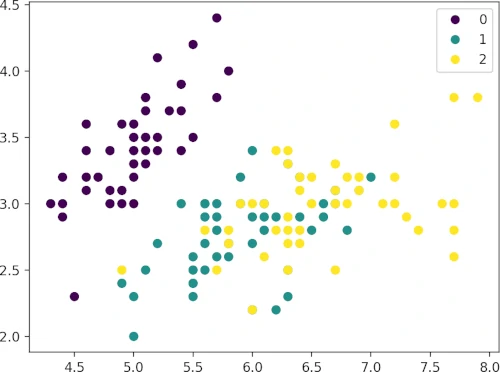### Create 3D scatter plot

Create a 3D scatter plot using three features from the iris dataset. To create a 3D plot, pass the argument
`projection="3d"` to the `Figure.add_subplot` function.

``````fig = plt.figure()
ax.scatter(df["sepal length (cm)"], df["sepal width (cm)"], df["petal length (cm)"], c=df["target"])
ax.set_xlabel('sepal length (cm)')
ax.set_ylabel('sepal width (cm)')
ax.set_zlabel('petal length (cm)')
plt.legend(s.legend_elements(), list(set(df["target"])))
plt.show()
``````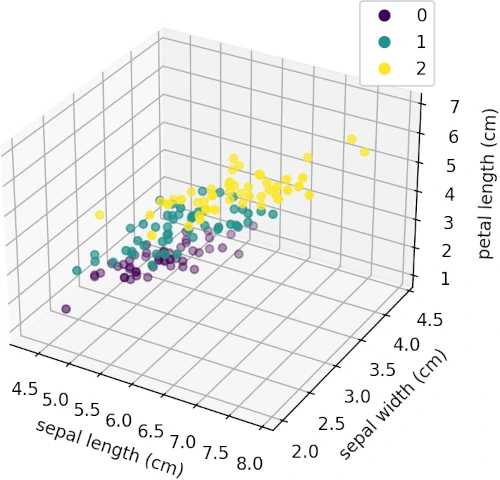## Enhance your skills with courses on Python

If you have any questions, comments or recommendations, please email me at reneshbe@gmail.com